# 5 Behavioral Analysis

We are now ready to analyze and plot the behavioral data. Let’s start with a quick recap of the data and tasks we have:

The day sorting task (Figure 1D) was performed in front of a computer screen. The 20 event images from the day learning task were presented on the screen in a miniature version. They were arranged in a circle around a central area displaying 4 rectangles. Participants were instructed to drag and drop all events of the same sequence into the same rectangle with a computer mouse. Participants freely chose which rectangle corresponded to which sequence as the sequence were not identifiable by any label and were presented in differing orders across mini-blocks during learning.

Thus, in analysis, we took the grouping as provided by the rectangles and assigned the four groups of events to the four days in a way that there was maximal overlap between actual days and sorted days. We found the best solution for this by trying all combinations in a preparation step.

In this task, participants saw a timeline ranging from 6 a.m. to midnight together with miniature versions of the five event images belonging to one sequence (Figure 1E). Participants were instructed to drag and drop the event images next to the timeline so that scene positions reflected the event times they had inferred in the day learning task. To facilitate precise alignment to the timeline, event images were shown with an outward pointing triangle on their left side, which participants were instructed to base their responses on.

Participants responses were read out from the logfiles of this task and converted to virtual hours. The data are saved in the text file including all behavioral data (virtem_behavioral_data.txt).

Let’s begin by loading the data into a tibble (dataframe) with the following columns:

• sub_id = subject identifier for all rows of this subject
• day = virtual day. There are 4 virtual days
• event = number of event in a day, i.e. order. Each day has 5 events.
• pic = picture identifier. Pictures were randomly assigned to events and days for each subject
• virtual_time = true virtual time of an event
• real_time = real time of an event
• memory_time = data from the timeline task, where participants indicated remembered (virtual) time of each event
• memory_order = remembered ordinal position of an event
• sorted_day = data from the day sorting task, where participants sorted all event pictures into the 4 days.
# load data from CSV
fname <- file.path(dirs$data4analysis, "behavioral_data.txt") col_types_list <- cols_only( sub_id = col_factor(), day = col_factor(), event = col_factor(), pic = col_integer(), virtual_time = col_double(), real_time = col_double(), memory_time = col_double(), memory_order = col_double(), sorted_day = col_integer() ) beh_data <- as_tibble(read_csv(fname, col_types = col_types_list)) head(beh_data) Table 5.1: sub_iddayeventpicvirtual_timereal_timememory_timememory_ordersorted_day 3111129.257.819.4511 31122011.8 23.4 11.5 21 3113214.8 42.2 14.5 31 3114816.2 51.6 16.5 41 31151918.2 64.1 19 51 312119.5 9.389.5312 ## 5.1 Sorting Task For analysis of the sorting task, we took the grouping of event images as provided by the participants and assigned them to the four sequences to ensure maximal overlap between actual and sorted sequence memberships. While the assignment of groupings to sequences is unambiguous when performance is, as in our sample, high, this procedure is potentially liberal at lower performance levels. We then calculated the percentage of correctly sorted event images (Figure 2A). ### Calculate percentage of correct responses So to calculate participants’ accuracy in this task we need to figure out how often the true day label matches the label of the quadrant into which an event was sorted. # calculate the number and percentages of correct sorts day_sorting <- beh_data %>% group_by(sub_id) %>% summarise( n_correct = sum(day == sorted_day), prcnt_correct = sum(day == sorted_day)/(n_days*n_events_day)*100, group = as.factor(1), .groups = "drop" ) # print a simple summary of descriptive statistics summary(day_sorting$prcnt_correct)
##    Min. 1st Qu.  Median    Mean 3rd Qu.    Max.
##   40.00   75.00   95.00   86.43  100.00  100.00

Results of the sorting task: 86.43 ± 16.82 mean±standard deviation of correct sorts

### Plot sorting performance

# raincloud plot
f2a <- ggplot(day_sorting,aes(x=1,y=prcnt_correct, fill = group, colour = group)) +
# plot the violin
gghalves::geom_half_violin(position=position_nudge(0.1),
side = "r", fill=ultimate_gray, color= NA) +
# single subject data points with horizontal jitter
geom_point(aes(x = 1-.2), alpha = 0.7,
shape=16, colour=ultimate_gray, size = 1,
position = position_jitter(width = .1, height = 0)) +
# box plot of distribution
geom_boxplot(width = .1, colour = "black") +
# add plot of mean and SEM
stat_summary(fun = mean, geom = "point", size=1, shape = 16,
position = position_nudge(.1), colour = "black") +
stat_summary(fun.data = mean_se, geom = "errorbar",
position = position_nudge(.1), colour = "black", width = 0, size = 0.5) +
# edit axis labels and limit
ylab('% correct') + ylim(0, 100) +
# make it pretty
theme_cowplot() + theme(legend.position = "none",
axis.ticks.x = element_blank(), axis.text.x = element_blank()) +
# change color
scale_color_manual(values = ultimate_gray) +
scale_fill_manual(values = ultimate_gray) +
guides(color=FALSE, fill=FALSE)
f2a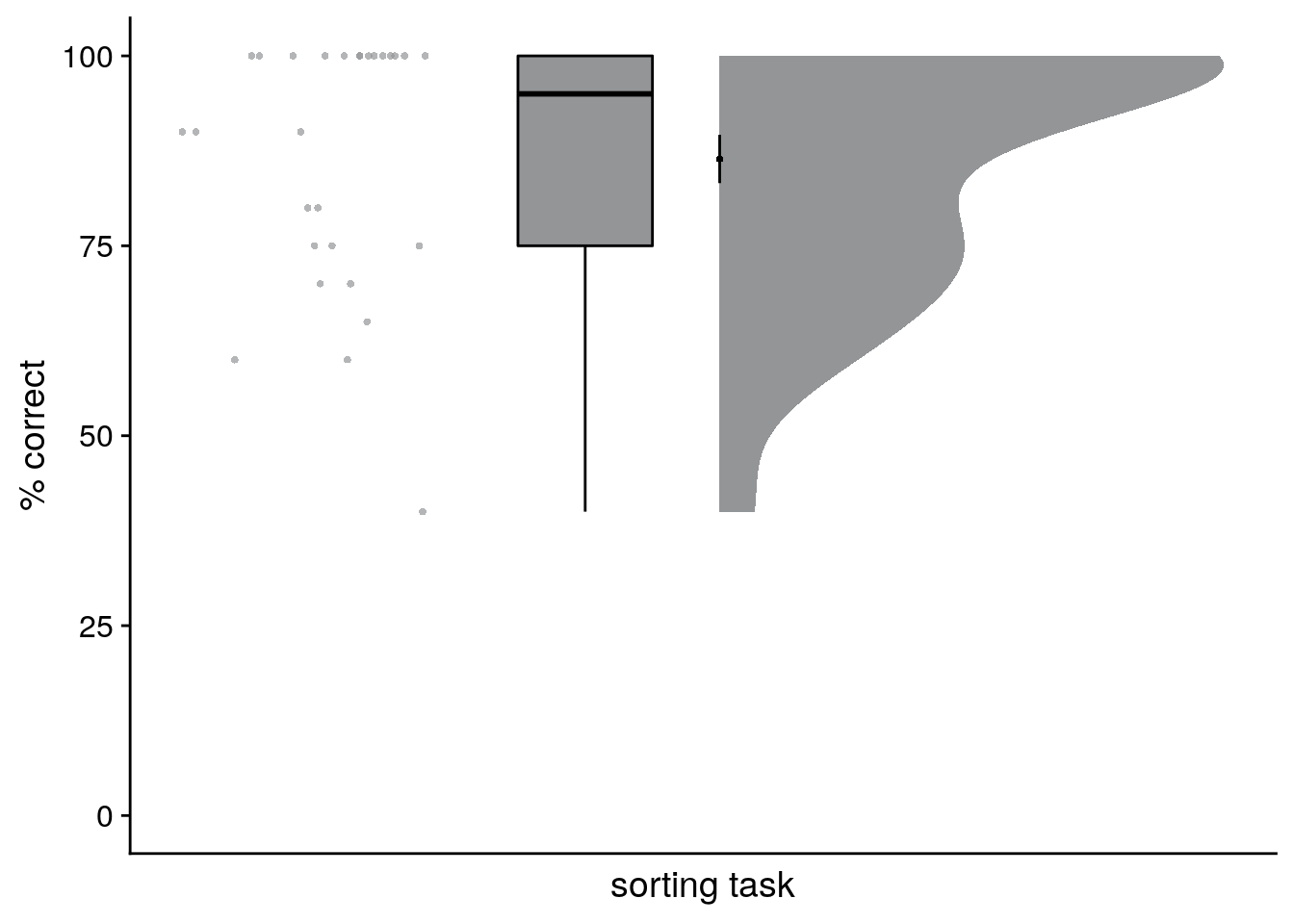### Visualize the raw data

First, let’s get an overview of participants’ behavior in this task. For this, we plot the responses from the timeline task for all participants. In these plots, each row is one virtual day. The circles along the gray lines represent the true virtual times that participants were supposed to learn. For each event on each day, we then add a full plot of the behavior. This includes the single-subject data points (colored circles), their mean and standard error across subjects (black circle and line) as well as the boxplot and kernel density plot of the distribution.

# reduce data frame to the times specified by the design
design_temp_struct <- beh_data %>% group_by(day, event) %>%
summarise(virtual_time = unique(virtual_time), .groups = "drop")

# raincloud plot
f2b <- ggplot(beh_data, aes(x=day,y=memory_time,
fill = virtual_time, colour = virtual_time,
group=paste(day, event, sep = "_"))) +
gghalves::geom_half_violin(position = "identity", side = "r", color = NA) +
# plot the violin
#geom_flat_violin(position = position_nudge(x = 0.1, y = 0),adjust = 1, trim = TRUE) +
# single subject data points with horizontal jitter
geom_point(aes(x = as.numeric(day)-0.25, y = memory_time, fill = virtual_time), alpha = 0.7,
position = position_jitter(width = .05, height = 0),
shape=16, size = 1) +
# box plot of distribution
geom_boxplot(position = position_nudge(x = -0.1, y = 0), aes(x = day, y = memory_time),
width = .05, colour = "black", outlier.shape = NA) +
# add plot of mean and SEM
stat_summary(fun = mean, geom = "point", size = 1, colour = "black", shape = 16) +
stat_summary(fun.data = mean_se, geom = "errorbar",
colour = "black", width = 0, size = 0.5)+
# plot point and line for true virtual times
geom_line(data = design_temp_struct, aes(x = day, y = virtual_time, group = day),
position = position_nudge(-0.45), size = 1, colour = ultimate_gray)+
geom_point(data = design_temp_struct, aes(x = day, y = virtual_time, fill = virtual_time),
position = position_nudge(-0.45), size = 2, color = ultimate_gray, stroke = 0.5, shape = 21) +
ylab('virtual time') + xlab('sequence') +
scale_y_continuous(limits = c(6, 24), breaks=seq(6,24,4)) +
scico::scale_fill_scico(begin = 0.1, end = 0.7, palette = "devon") +
scico::scale_color_scico(begin = 0.1, end = 0.7, palette = "devon") +
coord_flip() +
guides(color=guide_colorbar(title.position = "top", direction = "horizontal",
title = element_blank(),
barwidth = unit(20, "mm"), barheight = unit(3, "mm")),
fill=FALSE)+
theme_cowplot() + theme(text = element_text(size=10), axis.text = element_text(size=8),
legend.position = c(1,1), legend.justification = c(1,1))
f2b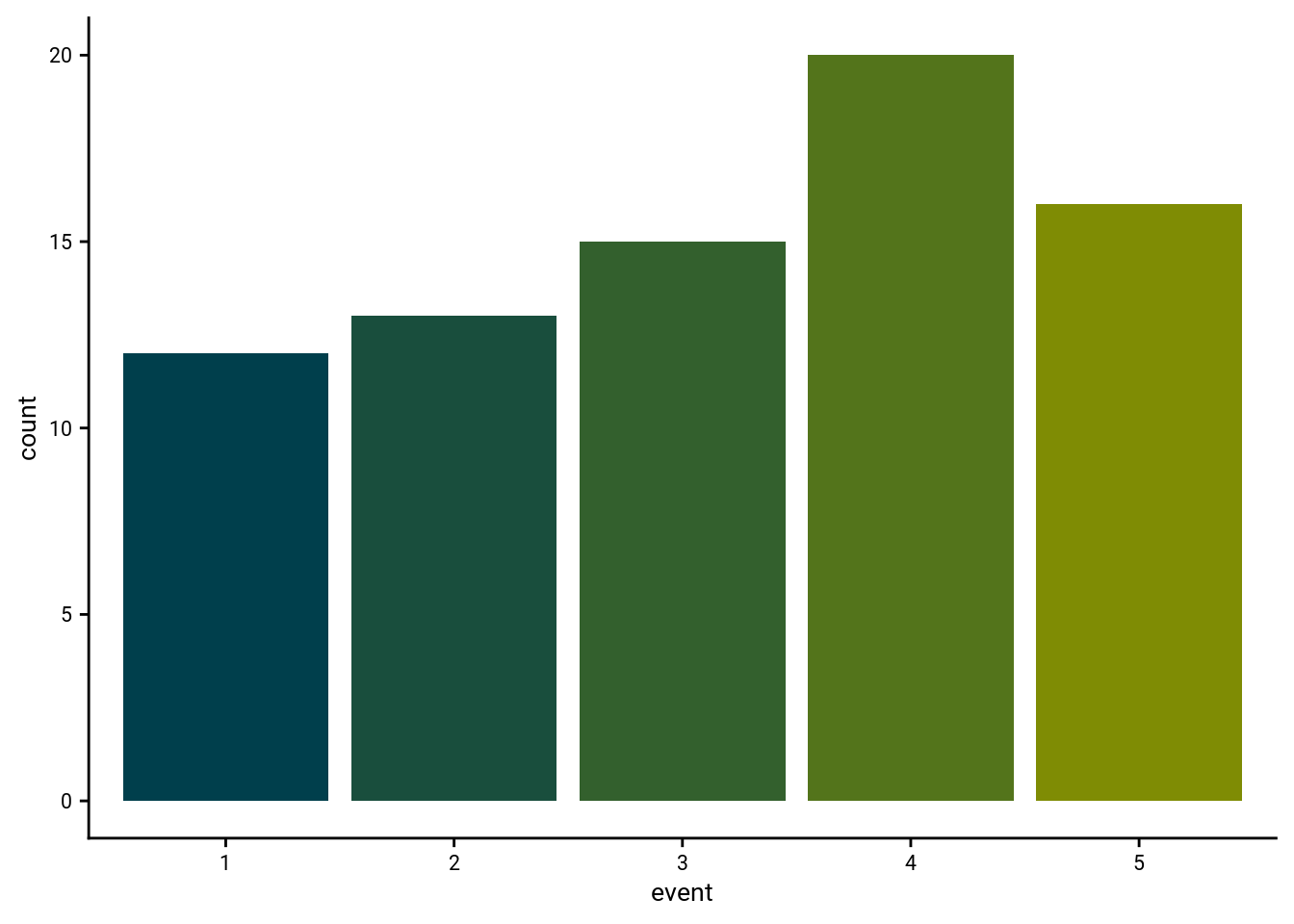### Accuracy of remembered times

We analyzed how well participants constructed the event times based on the day learning task. We quantified absolute errors across all events (Figure 2C) as well as separately for the five sequence positions (Figure 2D).

#### Average absolute error per participant

Now, let’s look at the average error per participant collapsed across all trials.

# calculate signed timeline error as difference between virtual time and response
beh_data <- beh_data %>%
mutate(timeline_error = virtual_time - memory_time)

# average absolute timeline error across subjects
timeline_group <- beh_data %>%
group_by(sub_id) %>%
summarise(
avg_error = mean(abs(timeline_error)),
.groups = "drop")

# print summary of the average error
summary(timeline_group$avg_error) ## Min. 1st Qu. Median Mean 3rd Qu. Max. ## 0.2377 0.5625 0.9093 0.9103 1.1221 2.0963 f2c <- ggplot(timeline_group, aes(x=1,y=avg_error), colour= time_colors, fill=time_colors) + # plot the violin gghalves::geom_half_violin(position=position_nudge(0.1), side = "r", fill=time_colors, color = NA) + # single subject data points with horizontal jitter geom_point(aes(x = 1-.2), alpha = 0.7, position = position_jitter(width = .1, height = 0), shape=16, colour=time_colors, size = 1)+ # box plot of distribution geom_boxplot(width = .1, fill=time_colors, colour = "black", outlier.shape = NA) + # add plot of mean and SEM stat_summary(fun = mean, geom = "point", size = 1, shape = 16, position = position_nudge(.1), colour = "black") + stat_summary(fun.data = mean_se, geom = "errorbar", position = position_nudge(.1), colour = "black", width = 0, size = 0.5)+ # edit axis labels ylab('absolute error') + xlab('timeline task') + guides(color=FALSE, fill=FALSE)+ theme_cowplot() + theme(legend.position = "none", axis.text.x = element_blank(), axis.ticks.x = element_blank()) f2c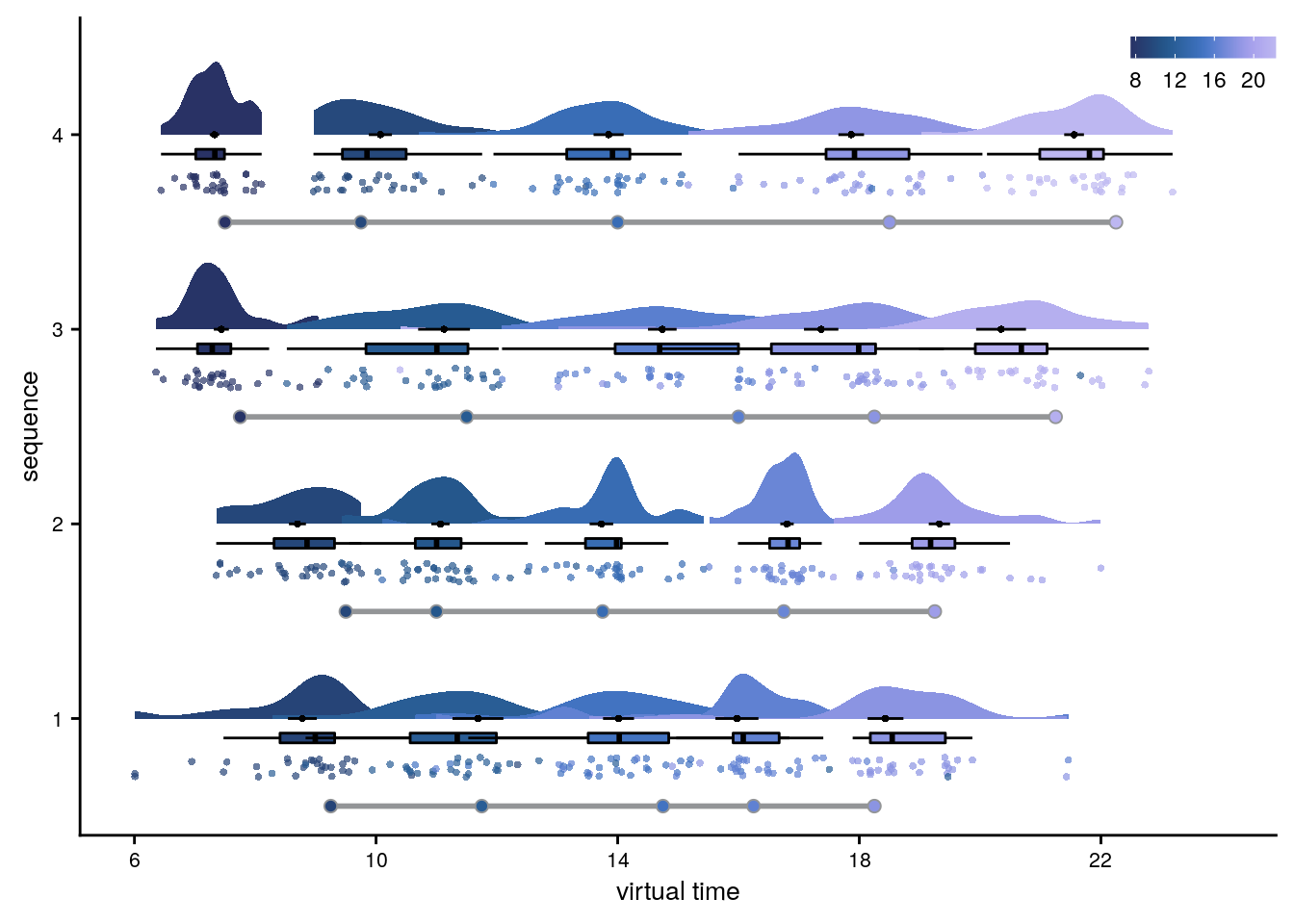Timeline task: 0.91 ± 0.47 mean±standard deviation of average absolute errors. #### Event-wise average absolute errors We can also aggregate the data across days by group the events as a function of event position (i.e. the order). Let’s look at the absolute errors in the timeline task for the events at positions 1-5, averaged across days. # calculate mean absolute error per event for each subject timeline_per_event <- beh_data %>% group_by(sub_id, event) %>% summarise( mean_abs_error =mean(abs(timeline_error)), .groups = "drop") # raincloud plot f2d <- ggplot(timeline_per_event,aes(x=event,y=mean_abs_error, fill = event)) + gghalves::geom_half_violin(position=position_nudge(0.1), side = "r", colour=NA) + geom_point(aes(x = as.numeric(event)-.2, y = mean_abs_error, colour=event), alpha = 0.7, position = position_jitter(width = .1, height = 0), shape=16, size = 1) + geom_boxplot(position = position_nudge(x = 0, y = 0), aes(x = event, y = mean_abs_error), width = .1, colour = "black", outlier.shape = NA, outlier.size = 1) + stat_summary(fun = mean, geom = "point", size=1, shape = 16, position = position_nudge(.1), colour = "BLACK") + stat_summary(fun.data = mean_se, geom = "errorbar", position = position_nudge(.1), colour = "BLACK", width = 0, size = 0.5)+ ylab('absolute error')+ xlab('event') + scale_colour_manual(values = event_colors, name="event position") + scale_fill_manual(values = event_colors) + guides(fill=FALSE, color=guide_legend(override.aes=list(fill=NA, alpha = 1, size=2))) + theme_cowplot() + theme(legend.position = "none") f2d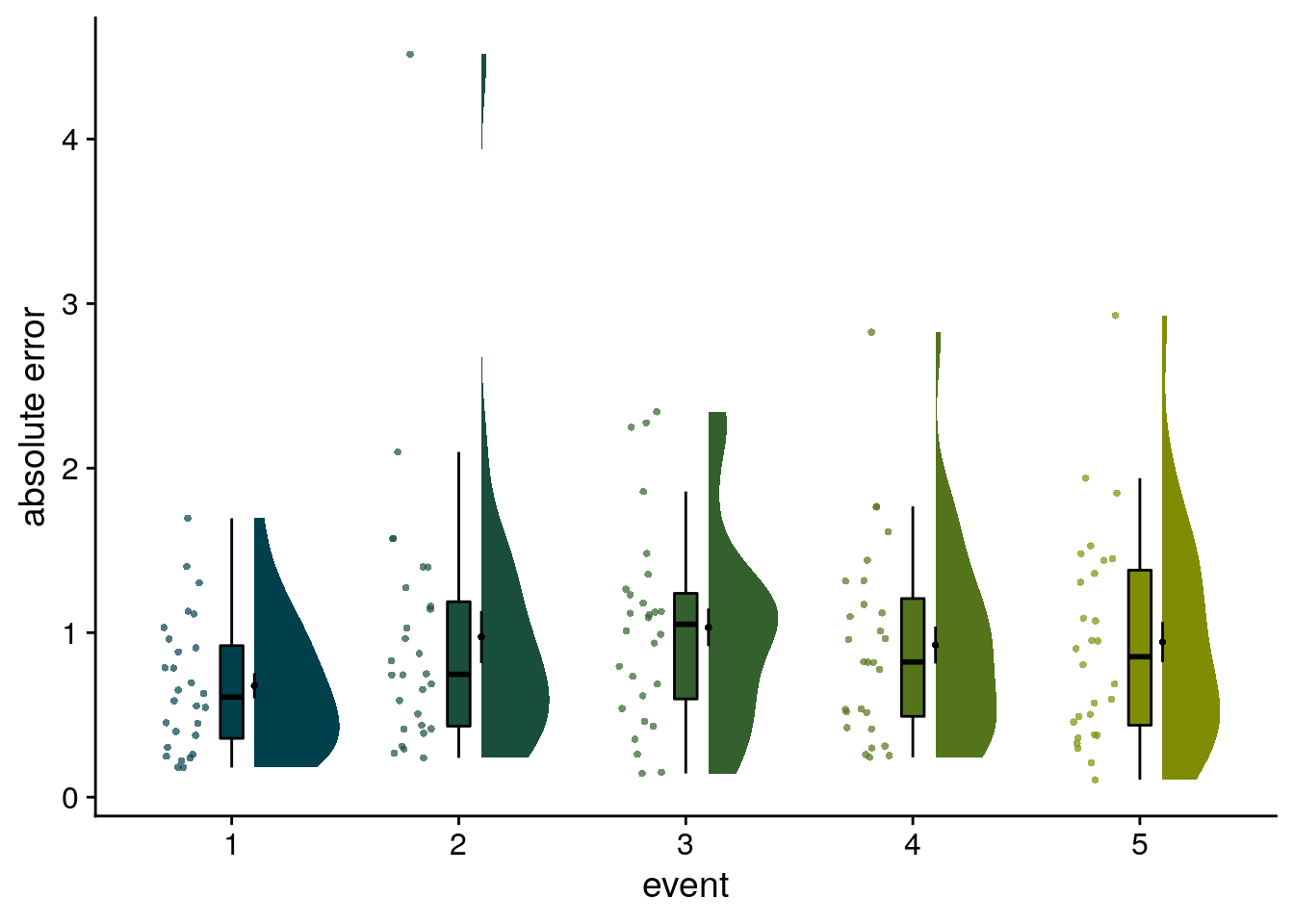### Time metrics underlying remembered times Further, using two approaches we tested whether virtual time drove participants’ responses rather than the sequence order or objectively elapsing time. While participants were asked to reproduce the virtual time, any of the other two factors could have an impact on their behavioral responses. If participants had, for example, only memorized the order of scenes in a given day, they would probably do reasonably well on the timeline task by distributing the scenes evenly along the timeline in the correct order. #### Summary Statistics For the summary statistics approach, we ran a multiple regression analysis for each participant with virtual time, sequence position (order), and real time since the first event of a day as predictors of responses in the timeline task. To test whether virtual time indeed explained participants’ responses even when competing for variance with order and real time, included in the model as control predictors of no interest, we compared the participant-specific t-values of the resulting regression coefficients against null distributions obtained from shuffling the remembered times against the predictors 10,000 times. We converted the resulting p-values to Z-values and tested these against zero using a permutation-based t-test (two-sided; α=0.05; 10,000 random sign-flips, Figure 2E). As a measure of effect size, we report Cohen’s d with Hedges’ correction and its 95% confidence interval as computed using the effsize-package106. set.seed(115) # set seed for reproducibility # do RSA using linear model and calculate z-score for model fit from permutations fit <- beh_data %>% group_by(sub_id) %>% # run the linear model do(z = lm_perm_jb(in_dat = ., lm_formula = "memory_time ~ virtual_time + event + real_time", nsim = n_perm)) %>% mutate(z = list(setNames(z, c("z_intercept", "z_virtual_time", "z_order", "z_real_time")))) %>% unnest_longer(z) %>% filter(z_id != "z_intercept") %>% mutate(z_id = factor(z_id, levels = c("z_virtual_time", "z_order", "z_real_time"))) # run group-level t-test for virtual time stats <- fit %>% filter(z_id =="z_virtual_time") %>% select(z) %>% paired_t_perm_jb (., n_perm = n_perm) # Cohen's d with Hedges' correction for one sample using non-central t-distribution for CI d<-cohen.d(d=(fit %>% filter(z_id =="z_virtual_time") %>% select(z))$z, f=NA, paired=TRUE, hedges.correction=TRUE, noncentral=TRUE)
stats$d <- d$estimate
stats$dCI_low <- d$conf.int[]
stats$dCI_high <- d$conf.int[]

# print results
huxtable(stats) %>% theme_article()
(#tab:multiple regression timeline)
estimatestatisticp.valuep_permparameterconf.lowconf.highmethodalternativeddCI_lowdCI_high
2.4410.63.82e-110.0001271.972.91One Sample t-testtwo.sided1.951.382.7
# raincloud plot of the results
f2e <- ggplot(fit, aes(x=z_id, y=z, fill = z_id, colour = z_id)) +
# plot the violin
gghalves::geom_half_violin(position=position_nudge(0.1), side = "r", colour=NA) +
# single subject data points with horizontal jitter
geom_point(aes(x = as.numeric(z_id)-.2, y = z, colour = z_id), alpha = 0.7,
position = position_jitter(width = .1, height = 0), shape = 16, size = 1) +
geom_boxplot(position = position_nudge(x = 0, y = 0), aes(x = z_id, y = z),
width = .1, colour = "black", outlier.shape = NA, outlier.size = 2) +
stat_summary(fun = mean, geom = "point", size=1, shape = 16,
position = position_nudge(.1), colour = "BLACK") +
stat_summary(fun.data = mean_se, geom = "errorbar",
position = position_nudge(.1), colour = "BLACK", width = 0, size = 0.5) +
ylab('Z multiple regression')+xlab('time metric') +
scale_x_discrete(labels = c("virt. time", "order", "real time")) +
coord_cartesian(ylim = c(-2,4.5))+
scale_color_manual(labels = c("virtual time", "order", "real time"),values=time_colors) +
scale_fill_manual(labels = c("virtual time", "order", "real time"),values=time_colors) +
guides(fill=FALSE, color=guide_legend(override.aes=list(fill=NA, alpha = 1, size=2),
title = element_blank(), direction ="vertical",
title.position = "bottom")) +
annotate(geom = "text", x = 1, y = 4.2, label = "***", hjust = 0.5, size = 8/.pt, family=font2use) +
theme_cowplot() + theme(text = element_text(size=8), axis.text = element_text(size=8),
legend.position = c(1,1), legend.justification = c(1,1),
legend.spacing.x = unit(0, 'mm'), #legend.spacing.y = unit(2, 'mm'),
legend.key.size = unit(3,"mm"),
legend.box.margin = margin(0,0,0,0,"mm"), legend.background = element_rect(size=0))
f2e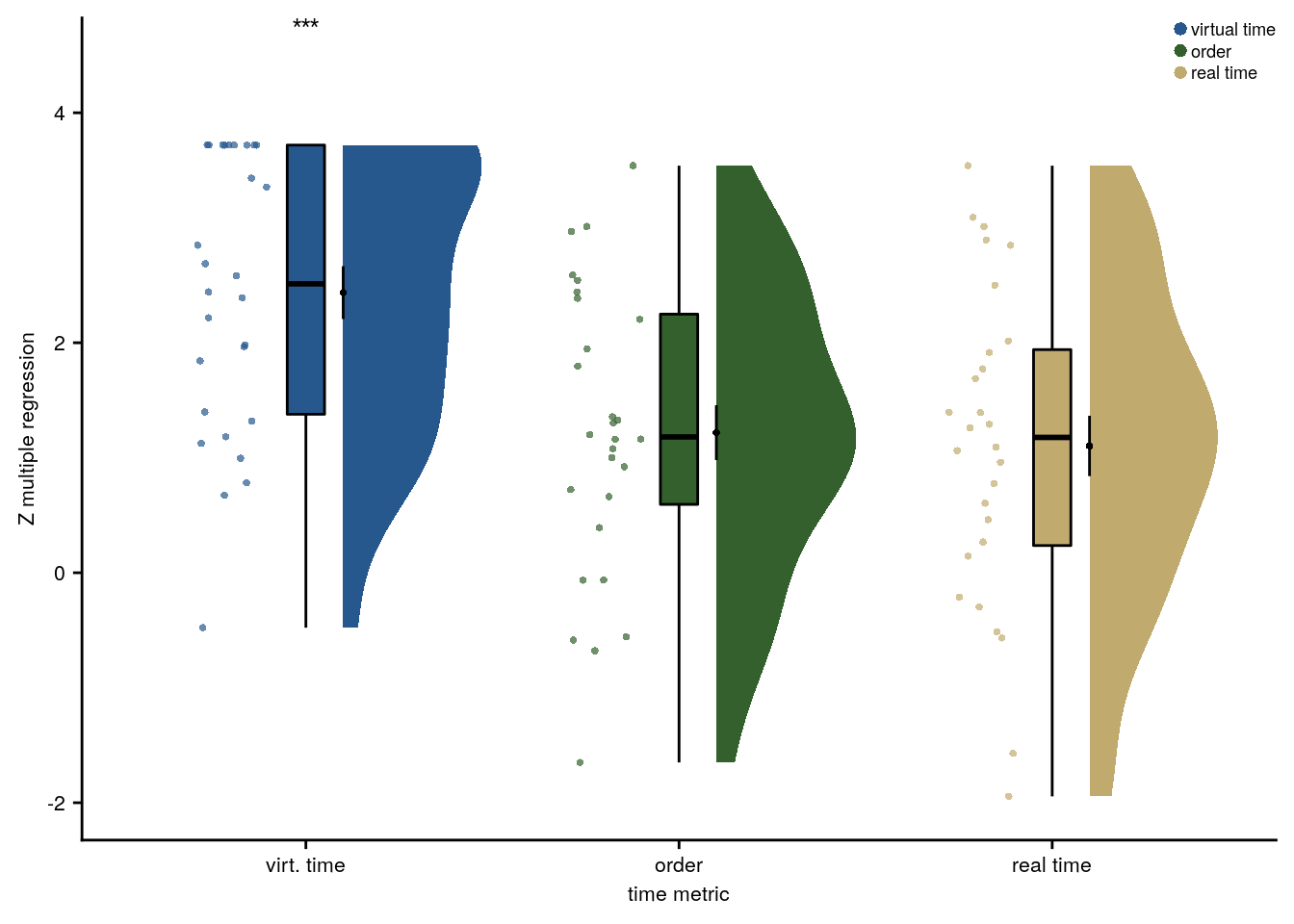Summary Statistics: t-test against 0 for virtual time when order and real time are in the model
t27=10.62, p=0.000, d=1.95, 95% CI [1.38, 2.70]

#### Linear mixed effects

A potentially more elegant way of testing the above is to use linear mixed effects models. However, drawing statistical inferences from these data is less straight forward and care needs to be taken with respect to the precise hypotheses that are tested.

Second, we addressed this question using linear mixed effects modeling. Here, we included the three z-scored time metrics as fixed effects. Starting from a maximal random effect structure (Barr et al., 2013), we simplified the random effects structure to avoid convergence failures and singular fits. The final model included random intercepts and random slopes for virtual time for participants. The model results are visualized by dot plots showing the fixed effect parameters with their 95% confidence intervals (Figure 2F) and marginal effects (Figure 2G) estimated using the ggeffects package (Lüdecke, 2018). To assess the statistical significance of virtual time above and beyond the effects of order and real time, we compared this full model to a nested model without the fixed effect of virtual time, but including order and real time, using a likelihood ratio test. Supplemental Table 1 provides an overview of the final model and the model comparison.

The main point we want to make is that virtual time explains variance above and beyond order. To test this, we run the full LMM and a reduced version of the model without virtual time. These two models are then compared using a likelihood ratio test to obtain a p-value.

Following Barr et al. (2013), we want to implement a maximal random effect structure. However, for the models to converge and to avoid singular fits, we have to simplify the random effects structure to include only random intercepts for each subject and a random slope for the effect of virtual time for each subject. Barr et al. (2013, p. 275) “propose the working assumption that it is not essential for one to specify random effects for control predictors to avoid anti-conservative inference, as long as interactions between the control predictors and the factors of interest are not present in the model”. However, “in the common case where one is interested in a minimally anti-conservative evaluation of the strength of evidence for the presence of an effect, our results indicate that keeping the random slope for the predictor of theoretical interest is important” (Barr et al., 2013, p. 276). Thus, we keep the random slopes for virtual time in the model.

More specifically, we follow these steps:

• we start with a model that includes random intercepts and slopes for all 3 fixed effects for each subject. This results in a singular fit
• Thus, we drop the random effects of real time and order.

We do not incorporate random intercepts for the individual events as this sampling unit cannot be dissociated from the predictors. See the description of the exception by Brauer & Curtin (Psych Methods, 2018, p. 13).

# center the predictor variables (set scale to true for z-score)
beh_data <- beh_data %>%
group_by(sub_id) %>%
mutate(
order_z = scale(as.numeric(event), scale = TRUE),
virtual_time_z = scale(virtual_time, scale = TRUE),
real_time_z = scale(real_time, scale = TRUE)
) %>%
ungroup()

# set RNG
set.seed(27)

# define the full model with all 3 time metrics as fixed effects and
# by-subject random intercepts and random slopes for each time metric --> singular fit
formula <- "memory_time ~ virtual_time_z + order_z + real_time_z + (1 + virtual_time_z + order_z + real_time_z | sub_id)"
lmm_full <- lme4::lmer(formula, data = beh_data,
REML = FALSE, control=lmerControl(optCtrl=list(maxfun=20000)))
## boundary (singular) fit: see ?isSingular
# remove random slopes for the fixed effects of non-interest (order and real time)
# by-subject random intercepts and random slopes for virtual time
set.seed(212) # set seed for reproducibility
formula <- "memory_time ~ virtual_time_z + order_z + real_time_z + (1 + virtual_time_z | sub_id)"
lmm_full <- lme4::lmer(formula, data = beh_data,
REML = FALSE, control=lmerControl(optCtrl=list(maxfun=20000)))
summary(lmm_full)
## Linear mixed model fit by maximum likelihood  ['lmerMod']
## Formula: memory_time ~ virtual_time_z + order_z + real_time_z + (1 + virtual_time_z |      sub_id)
##    Data: beh_data
## Control: lmerControl(optCtrl = list(maxfun = 20000))
##
##      AIC      BIC   logLik deviance df.resid
##   1940.0   1974.6   -962.0   1924.0      552
##
## Scaled residuals:
##     Min      1Q  Median      3Q     Max
## -7.3874 -0.4034  0.0429  0.4259  7.8191
##
## Random effects:
##  Groups   Name           Variance Std.Dev. Corr
##  sub_id   (Intercept)    0.04928  0.2220
##           virtual_time_z 0.02742  0.1656   0.23
##  Residual                1.75541  1.3249
## Number of obs: 560, groups:  sub_id, 28
##
## Fixed effects:
##                Estimate Std. Error t value
## (Intercept)    14.01002    0.06996 200.252
## virtual_time_z  3.06932    0.25997  11.807
## order_z         1.66763    0.43023   3.876
## real_time_z    -0.33226    0.47331  -0.702
##
## Correlation of Fixed Effects:
##             (Intr) vrtl__ ordr_z
## virtul_tm_z  0.017
## order_z      0.000 -0.112
## real_time_z  0.000 -0.426 -0.841
# tidy summary of the fixed effects that calculates 95% CIs
lmm_full_bm <- broom.mixed::tidy(lmm_full, effects = "fixed", conf.int=TRUE, conf.method="profile")
## Computing profile confidence intervals ...
# tidy summary of the random effects
lmm_full_bm_re <- broom.mixed::tidy(lmm_full, effects = "ran_pars")

To test whether virtual time is relevant to explaining the data even when order and real time are in the model, we compare the full model defined above against a reduced model. In this reduced model, we do not include the fixed effect of virtual time. We compare the full against the nested (reduced) model using a likelihood ratio test.

# to test for significance let's by comparing the likelihood against a simpler model.
# Here, we drop the effect of virtual time and run an ANOVA. See e.g. Bodo Winter tutorial
# this is the current best way of testing the effect of virtual time on behavior
formula <- "memory_time ~ order_z + real_time_z + (1 + virtual_time_z | sub_id)" # random intercepts for each subject and random slopes for virtual time
set.seed(213) # set seed for reproducibility
lmm_no_vir_time <- lme4::lmer(formula, data = beh_data, REML = FALSE, control=lmerControl(optCtrl=list(maxfun=20000)))

# because the model fails to converge with a warning that the scaled gradient at the fitted (RE)ML estimates
# is large, we restart the model as described here (https://rdrr.io/cran/lme4/man/troubleshooting.html)
# obtaining consistent results (with no warning) suggests a false positive
lmm_no_vir_time <- update(lmm_no_vir_time, start=getME(lmm_no_vir_time, "theta"))

# run the ANOVA
lmm_aov <- anova(lmm_no_vir_time, lmm_full)
lmm_aov
Table 5.2:
nparAICBIClogLikdevianceChisqDfPr(>Chisq)
72.05e+032.08e+03-1.02e+032.04e+03
81.94e+031.97e+03-962       1.92e+0311614.88e-27

Mixed Model: Fixed effect of virtual time time with order and real time in the model of remembered times $$\chi^2$$(1)=115.95, p=0.000

Make mixed model summary table that includes overview of fixed and random effects as well as the model comparison to the nested (reduced) model.

fe_names <- c("intercept", "virtual time", "order", "real time")
re_groups <- c(rep("participant",3), "residual")
re_names <- c("intercept", "virtual time (SD)", "correlation random intercepts and random slopes", "SD")

lmm_hux <- make_lme_huxtable(fix_df=lmm_full_bm,
ran_df = lmm_full_bm_re,
aov_mdl = lmm_aov,
fe_terms =fe_names,
re_terms = re_names,
re_groups = re_groups,
lme_form = gsub(" ", "", paste0(deparse(formula(lmm_full)),
collapse = "", sep="")),
caption = "Mixed Model: Virtual time explains constructed times with order and real time in the model")
## Warning: select_vars() is deprecated as of dplyr 0.8.4.
## Please use tidyselect::vars_select() instead.
## This warning is displayed once every 8 hours.
## Call lifecycle::last_warnings() to see where this warning was generated.
# convert the huxtable to a flextable for word export
stable_lme_memtime_time_metrics <- convert_huxtable_to_flextable(ht = lmm_hux)

# print to screen
theme_article(lmm_hux)
fixed effects term estimate SE t-value 14.010019 0.069962 200.25 13.868056 14.151981 3.069324 0.259967 11.81 2.558874 3.579774 1.667630 0.430230 3.88 0.822785 2.512476 -0.332261 0.473306 -0.70 -1.261696 0.597173 0.221991 0.232089 0.165592 1.324919 2053.90 -1019.95 1939.95 -961.98 115.95 1 4.88e-27

To visualize the model, we use the confidence intervals to create dot plots for the model coefficients. Further, we estimate marginal means for each fixed effect while holding the other parameters constant. We do this for the quartiles (including minimum and maximum values).

# make the time metrics a factor
lmm_full_bm <- lmm_full_bm %>%
mutate(term=as.factor(term) %>%
factor(levels = c("virtual_time_z", "order_z", "real_time_z")))

# dot plot of Fixed Effect Coefficients with CIs
f2f <- ggplot(data = lmm_full_bm[2:4,], aes(x = term, color = term)) +
geom_hline(yintercept = 0, colour="black", linetype="dotted") +
geom_errorbar(aes(ymin = conf.low, ymax = conf.high, width = NA), size = 0.5) +
geom_point(aes(y = estimate), size = 1, shape = 16) +
scale_fill_manual(values = time_colors) +
scale_color_manual(values = time_colors, labels = c("Virtual Time", "Order", "Real Time")) +
labs(#title = "Fixed Effect Coefficients",
x = element_blank(), y="fixed\neffect estimate",color = "Time Metric") +
theme_cowplot() +
#coord_fixed(ratio = 3) +
theme(plot.title = element_text(hjust = 0.5), axis.text.x=element_blank()) +
guides(color=FALSE, fill=FALSE)+
annotate(geom = "text", x = 1, y = 3.8, label = "***", hjust = 0.5, size = 8/.pt, family=font2use)
f2f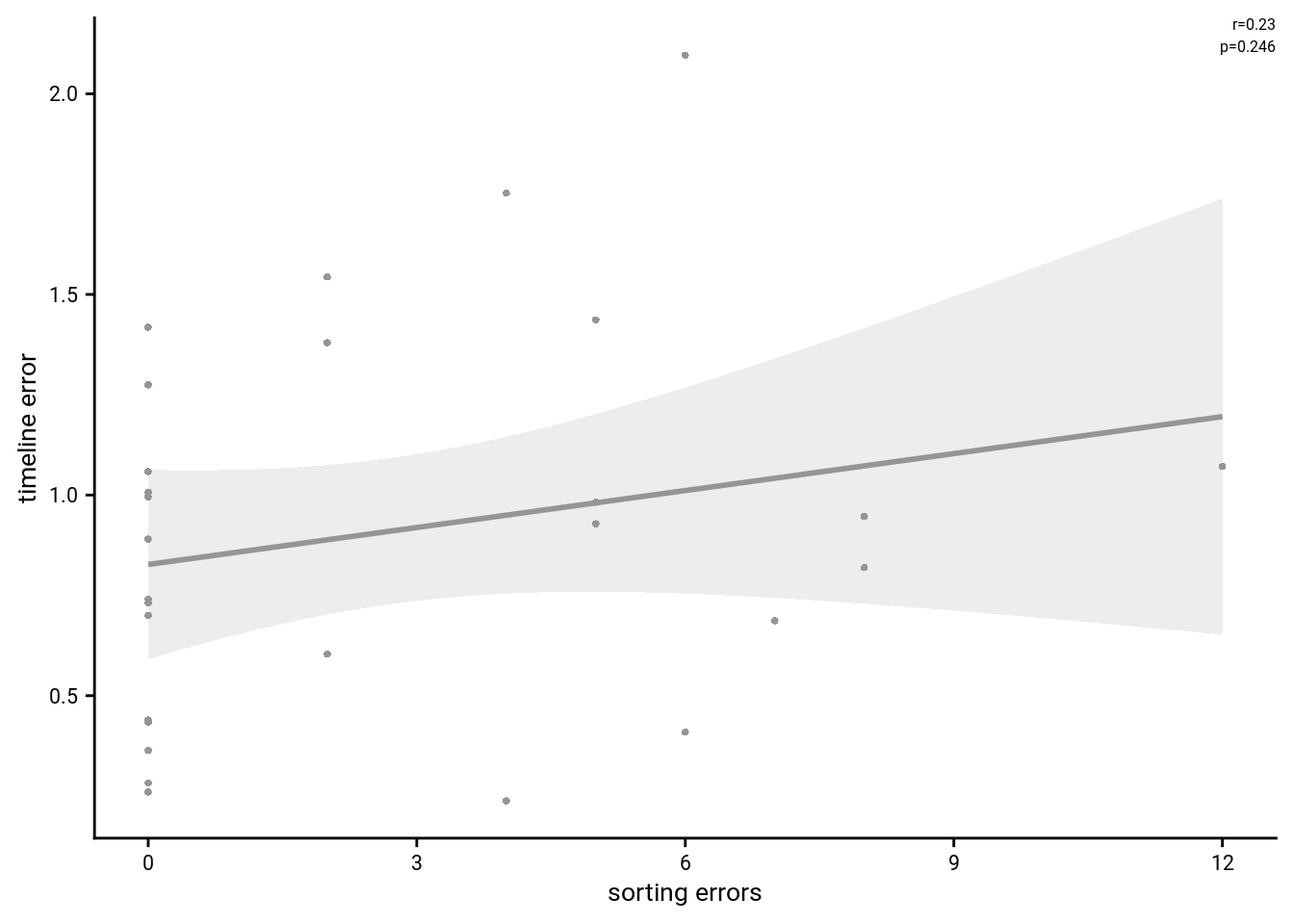# estimate marginal means for each model term by omitting the terms argument
lmm_full_emm <- ggeffects::ggpredict(lmm_full, ci.lvl = 0.95) %>% get_complete_df
# convert the group variable to a factor to control the order of facets below
lmm_full_emm$group <- factor(lmm_full_emm$group, levels = c("virtual_time_z", "order_z", "real_time_z"))

# plot marginal means
f2g <- ggplot(data = lmm_full_emm, aes(color = group)) +
geom_line(aes(x, predicted)) +
geom_ribbon(aes(x, ymin = conf.low, ymax = conf.high, fill = group), alpha = .3, linetype=0) +
scale_color_manual(values = time_colors, name=element_blank(),
labels = c("virtual time", "order", "real time")) +
scale_fill_manual(values = time_colors, labels = c("Virtual Time", "Order", "Real Time")) +
ylab('estimated\nmarginal means') +
xlab('z time metric') +
scale_y_continuous(breaks = c(9, 14, 19), labels = c(9, 14, 19)) +
guides(fill = FALSE, color=FALSE) +
theme_cowplot() +
theme(plot.title = element_text(hjust = 0.5), strip.background = element_blank(),
strip.text.x = element_blank())
f2g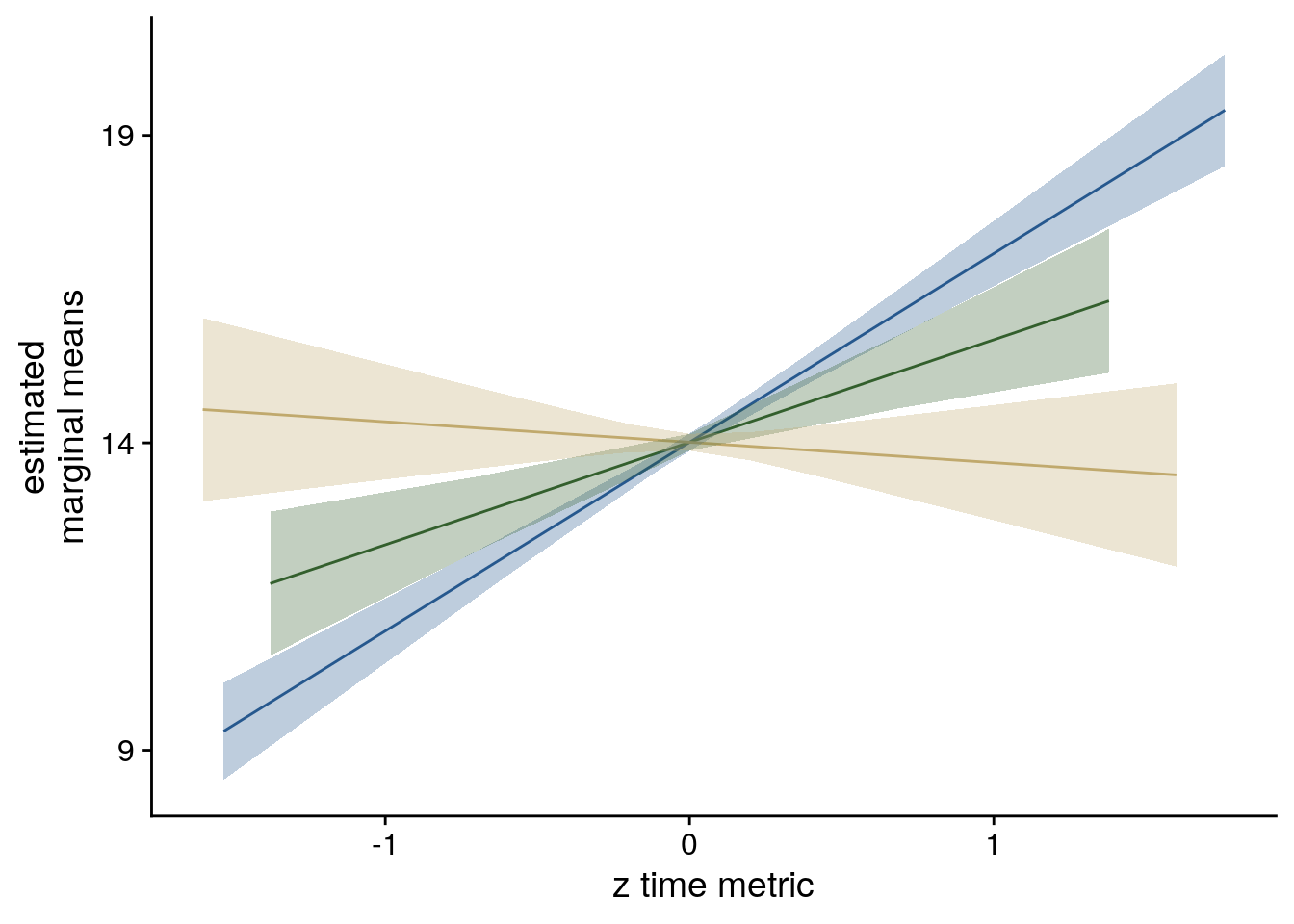##### LME model assumptions
lmm_diagplots_jb(lmm_full)• Absence of collinearity: The different time metrics are correlated. This correlation is slightly reduced by z-scoring the predictors. In any case, these correlations are inherent to the design and not really a problem as long as estimated coefficients are interpreted correctly. For more info, see e.g. this opinion piece.

## Compose Figure for Behavioral Data

This figure consists of the plots showing the results of the sorting task and the timeline task. It will probably be figure 2 of the manuscript.

layout = "
AABBBBBBDDDDDD
AABBBBBBDDDDDD
AABBBBBBDDDDDD
AABBBBBBDDDDDD
AABBBBBBDDDDDD
AABBBBBBDDDDDD
CCBBBBBBEEEEFF
CCBBBBBBEEEEFF
CCBBBBBBEEEEFF
CCBBBBBBEEEEGG
CCBBBBBBEEEEGG
CCBBBBBBEEEEGG"

f2 <- f2a + f2b + f2c + f2d + f2e + f2f + f2g +
plot_layout(design = layout, guides = "keep") &
theme(text = element_text(size=10, family=font2use),
axis.text = element_text(size=8),
legend.text=element_text(size=8),
legend.title=element_text(size=8)
) &
plot_annotation(theme = theme(plot.margin = margin(t=0, r=0, b=0, l=-5, unit="pt")),
tag_levels = 'A')

# save as png and pdf and print to screen
fn <- here("figures", "f2")
ggsave(paste0(fn, ".pdf"), plot=f2, units = "cm",
width = 17.4, height = 16, dpi = "retina", device = cairo_pdf)
ggsave(paste0(fn, ".png"), plot=f2, units = "cm",
width = 17.4, height = 16, dpi = "retina", device = "png")
f2Figure 2. Participants learn the temporal structure of the sequences relative to the virtual clock. A. Plot shows the percentage of correctly sorted event images in the sorting task. B. Constructed event times were assessed in the timeline task. Responses are shown separately for each of the five events (color coded according to true virtual time) of each of the four sequences (rows). Colored circles with gray outline at the bottom of each row show true event times. C, D. Mean absolute errors in constructed times (in virtual hours) are shown (C) averaged across events and sequences and (D) averaged separately for the five event positions. E. Z-values for the effects of different time metrics from participant-specific multiple regression analyses and permutation tests show that virtual time explained constructed event times with event order and real time in the model as control predictors. F. Likewise, parameter estimates and 95% confidence intervals for the fixed effects of the three time metrics from a linear mixed model indicate that virtual time relates to constructed event times beyond the effects of order and real time. G. Estimated marginal means (model predictions) illustrate the effects of the three time metrics. A-E. Circles are individual participant data; boxplots show median and upper/lower quartile along with whiskers extending to most extreme data point within 1.5 interquartile ranges above/below the upper/lower quartile; black circle with error bars corresponds to mean±S.E.M.; distributions show probability density function of data points. *** p<0.001

## 5.3 Generalization bias

If participants use structural knowledge about the sequences when constructing times of events, then we might expect biases in their behavior: Errors in constructed event times could be non-random. Specifically, when constructing the time of one specific event, participants could be biased in their response by the times of the events from other sequences at that sequence position. This would indicate that knowledge about the other sequences in generalized to influence specific mnemonic constructions, resulting in a bias.

### Quantify relative time of other events

To explore whether general time patterns bias the construction of event times, we assessed errors in remembered event times. Specifically, when constructing the time of one specific event, participants could be biased in their response by the times of the events from other sequences at that sequence position. For each event, we quantified the average time of events in the other sequences at the same sequence position (Figure 8A). For example, for the fourth event of the first sequence, we calculated the average time of the fourth events of sequences two, three and four.

To test for such a bias, we quantify, for each event, the relative time of the other events at that sequence position. We then calculate by how much virtual time each individual event time deviates from the average virtual time of the other events at that sequence position (e.g. the difference in virtual time for event 1 from sequence 1 compared to the average virtual time of events 1 from sequences 2-4). We do this so that positive values of this relative time measure indicate that the other events happened later than the event of interest.

# quantify the deviation in virtual time for each event relative to other events
# at the same sequence position
beh_data$rel_time_other_events<-NA for (i_day in 1:n_days){ for (i_event in 1:n_events_day){ # find the events at this sequence position from all four sequences curr_events <- beh_data[beh_data$event == i_event,]

# find the average virtual time of the other events at this sequence position
avg_vir_time_other_events <- curr_events %>%
filter(day != i_day) %>%
summarise(avg_vir_time = mean(virtual_time)) %>%
select(avg_vir_time) %>%
as.numeric(.)

# for the given event, store the relative time of the other events,
# as the difference in virtual time (positive values mean other events happen later)
event_idx <- beh_data$day==i_day & beh_data$event==i_event
beh_data$rel_time_other_events[event_idx] <- avg_vir_time_other_events - beh_data$virtual_time[event_idx]
}
}

### Test Generalization Bias

We then asked whether the deviation between the average time of other events and an event’s true virtual time was systematically related to signed errors in constructed event times. A positive relationship between the relative time of other events and time construction errors indicates that, when other events at the same sequence position are relatively late, participants are biased to construct a later time for a given event than when the other events took place relatively early.

The crucial test is then whether over- and underestimates of remembered time can be explained by this deviation measure.

# calculate timeline error so that positive numbers mean overestimates (later than true virtual time)
beh_data <- beh_data %>%
mutate(timeline_error=memory_time-virtual_time)

#### Summary statistics

In the summary statistics approach, we ran a linear regression model for each participant (Figure 8B, Supplemental Figure 8) and tested the resulting coefficients for statistical significance using the permutation-based procedures described above (Figure 8C).

In the summary statistics approach, we run a linear regression model for each participant and test the resulting coefficients against a permutation-based null distribution. The resulting z-scores are then tested against 0 on the group level.

# SUMMARY STATISTICS
set.seed(117) # set seed for reproducibility

# do RSA using linear model and calculate z-score for model fit from permutations
fit_beh_bias <- beh_data %>% group_by(sub_id) %>%
# run the linear model (also without permutation tests to get betas)
do(model = lm(timeline_error ~ rel_time_other_events, data=.),
z = lm_perm_jb(in_dat = .,
lm_formula = "timeline_error ~ rel_time_other_events",
nsim = n_perm)) %>%
# store beta estimates and their z-values
mutate(beta_rel_time_other_events = coef(summary(model))[2,"Estimate"],
t_rel_time_other_events = coef(summary(model))[2,"t value"],
z = list(setNames(z, c("z_intercept",  "rel_time_other_events")))) %>%
# get rid of intercept z-values and model column
unnest_longer(z) %>%
filter(z_id != "z_intercept") %>%
select (.,-c(model))

# run group-level t-tests on the RSA fits from the first level in aHPC for within-day
stats <- fit_beh_bias %>%
filter(z_id =="rel_time_other_events") %>%
select(z) %>%
paired_t_perm_jb (., n_perm = n_perm)

# Cohen's d with Hedges' correction for one sample using non-central t-distribution for CI
d<-cohen.d(d=fit_beh_bias$z, f=NA, paired=TRUE, hedges.correction=TRUE, noncentral=TRUE) stats$d <- d$estimate stats$dCI_low <- d$conf.int[] stats$dCI_high <- d$conf.int[] # print results huxtable(stats) %>% theme_article() Table 5.4: estimatestatisticp.valuep_permparameterconf.lowconf.highmethodalternativeddCI_lowdCI_high 1.385.321.3e-050.0001270.8491.92One Sample t-testtwo.sided0.9760.5521.48 Summary Statistics: t-test against 0 for generalization bias t27=5.32, p=0.000, d=0.98, 95% CI [0.55, 1.48] We observe a significant positive effect of the virtual time of other events at the same sequence position on remembered virtual time. That means that when other events at the same sequence position are later than a given event, participants are likely to overestimate the event time. Conversely, when the other events at this sequence position relatively early, participants underestimate. This demonstrates an across-sequence effect of virtual time. Virtual time is generalized across sequences to bias remembered times at similar sequence positions. To illustrate this effect, lets plot the data for one example subject (data from all subjects will be plotted below). For this, we pick a subject with an average fit. # pick average subject based on t-value of regression example_sub <- fit_beh_bias$sub_id[sort(fit_beh_bias$t_rel_time_other_events, decreasing = TRUE)[n_subs/2]==fit_beh_bias$t_rel_time_other_events]

f8b <- ggplot(beh_data%>%filter(sub_id==example_sub), aes(x=rel_time_other_events, y=timeline_error)) +
geom_smooth(method='lm', formula= y~x,
color=aHPC_colors["across_main"],
fill=aHPC_colors["across_main"])+
geom_point(size = 1, shape = 16) +
scale_x_continuous(breaks = c(-2.5, 0, 2.5), labels= c("-2.5", "0", "2.5")) +
xlab("relative time\nof other events") +
ylab("timeline error") +
theme_cowplot() +
theme(strip.background = element_blank(),
strip.text = element_blank(),
text = element_text(size=10, family=font2use),
axis.text = element_text(size=8))
f8b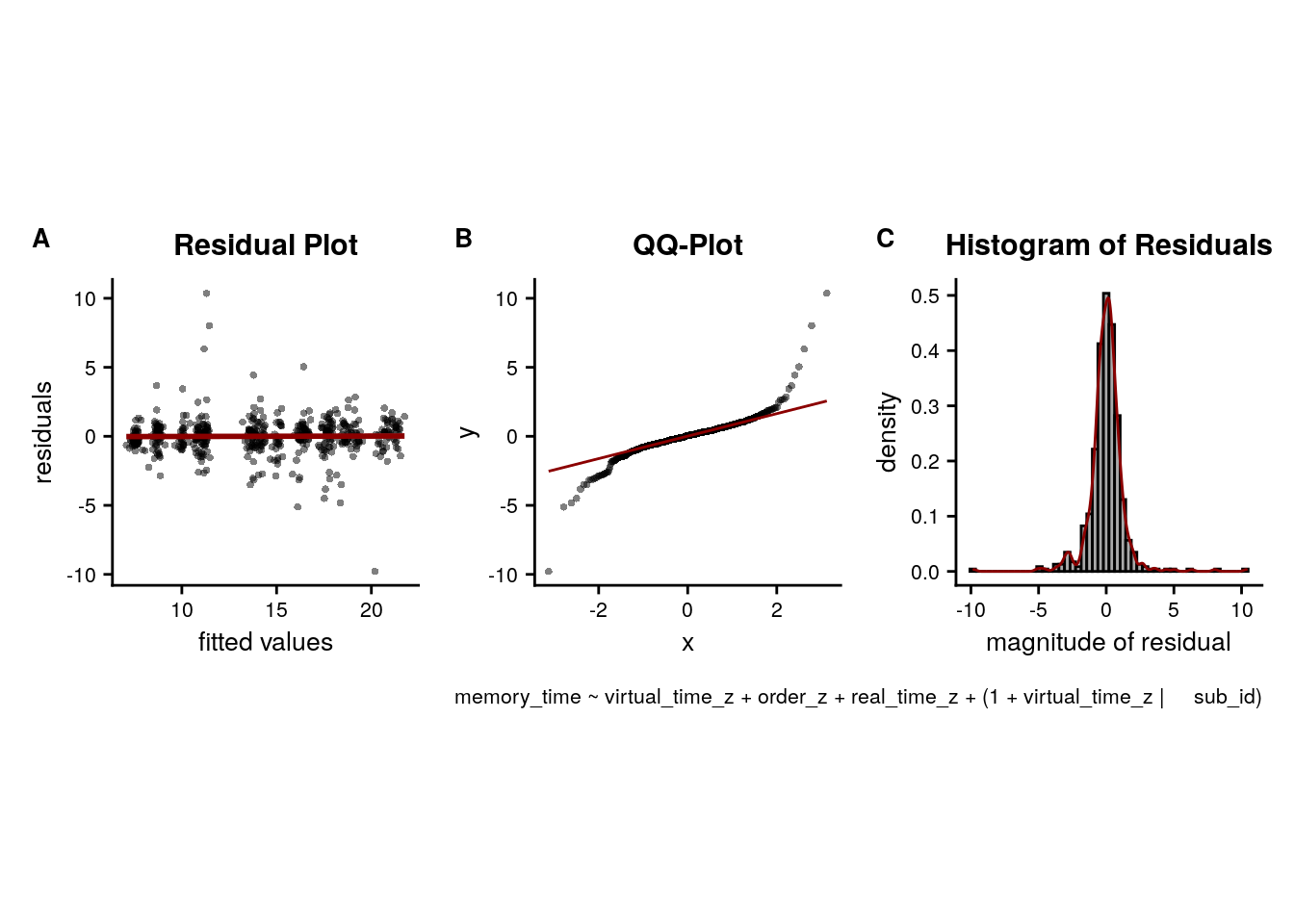To visualize the effect from the summary statistics approach on the group-level, we create a raincloud plot of the z-values from the linear model permutations for each subject.

# plot regression z-values
f8c<-ggplot(fit_beh_bias, aes(x=as.factor(1),y=z), colour= aHPC_colors["across_main"], fill=aHPC_colors["across_main"]) +
# plot the violin
gghalves::geom_half_violin(position=position_nudge(0.1),
side = "r", fill=aHPC_colors["across_main"], color = NA) +
geom_point(aes(x = 1-.2), alpha = 0.7,
position = position_jitter(width = .1, height = 0),
shape=16, colour=aHPC_colors["across_main"], size = 1)+
geom_boxplot(width = .1, fill=aHPC_colors["across_main"], colour = "black", outlier.shape = NA) +
stat_summary(fun = mean, geom = "point", size = 1, shape = 16,
position = position_nudge(.1), colour = "black") +
stat_summary(fun.data = mean_se, geom = "errorbar",
position = position_nudge(.1), colour = "black", width = 0, size = 0.5)+
ylab('Z regression') + xlab('generalization\n bias') +
annotate(geom = "text", x = 1, y = 4.2, label = "***", hjust = 0.5, size = 8/.pt, family=font2use) +
guides(color=FALSE, fill=FALSE)+
theme_cowplot() +
theme(axis.text.x = element_blank())
f8c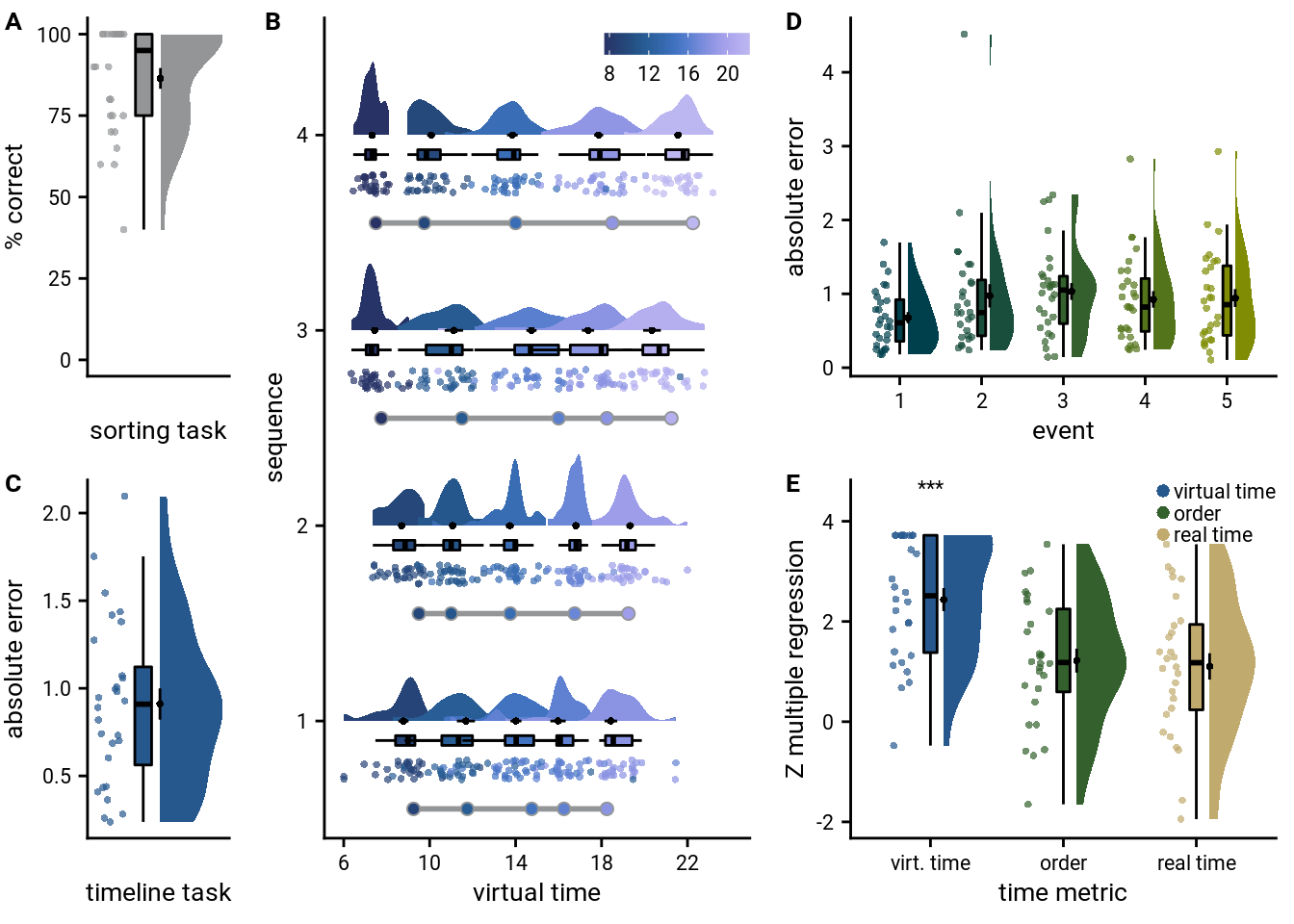#### Mixed Model

Further, we analyzed these data using the linear mixed model approach (Figure 8DE, Supplemental Table 13).

We also want to this effect using a linear mixed model. We use the maximal random effects structure with random intercepts and random slopes for participants.

Let’s fit the model and get tidy summaries.

# z-score the relative time of other events for each participant
beh_data <- beh_data %>%
group_by(sub_id) %>%
mutate(rel_time_other_events_z = scale(rel_time_other_events)) %>%
ungroup()

# Fit the model
set.seed(245) # set seed for reproducibility
lmm_full <- lme4::lmer("timeline_error ~ rel_time_other_events_z + (1+rel_time_other_events_z|sub_id)",
data=beh_data, REML=FALSE)

# tidy summary of the fixed effects that calculates 95% CIs
lmm_full_bm <- broom.mixed::tidy(lmm_full, effects = "fixed", conf.int=TRUE, conf.method="profile")
## Computing profile confidence intervals ...
# tidy summary of the random effects
lmm_full_bm_re <- broom.mixed::tidy(lmm_full, effects = "ran_pars")

Compare against a reduced model without the fixed effect of interest.

# fit reduced model
set.seed(248) # set seed for reproducibility
lmm_reduced <- lme4::lmer("timeline_error ~ 1 + (1+rel_time_other_events_z|sub_id)",
data=beh_data, REML=FALSE)
lmm_aov<-anova(lmm_full, lmm_reduced)
lmm_aov
Table 5.5:
nparAICBIClogLikdevianceChisqDfPr(>Chisq)
51.96e+031.98e+03-9741.95e+03
61.94e+031.97e+03-9651.93e+0317.912.32e-05

Mixed Model: Fixed effect of relative time of other events on timeline errors
$$\chi^2$$(1)=17.90, p=0.000

Make summary table.

fe_names <- c("intercept", "relative time other events")
re_groups <- c(rep("participant",3), "residual")
re_names <- c("intercept", "relative time other events (SD)", "correlation random intercepts and random slopes", "SD")

lmm_hux <- make_lme_huxtable(fix_df=lmm_full_bm,
ran_df = lmm_full_bm_re,
aov_mdl = lmm_aov,
fe_terms =fe_names,
re_terms = re_names,
re_groups = re_groups,
lme_form = gsub(" ", "", paste0(deparse(formula(lmm_full)),
collapse = "", sep="")),
caption = "Mixed Model: Behavioral generalization bias")

# convert the huxtable to a flextable for word export
stable_lme_beh_gen_bias <- convert_huxtable_to_flextable(ht = lmm_hux)

# print to screen
theme_article(lmm_hux)
fixed effects term estimate SE t-value -0.352481 0.069962 -5.04 -0.494444 -0.210518 0.337262 0.067360 5.01 0.200579 0.473945 0.220016 -0.114173 0.183681 1.331485 1958.57 -974.29 1942.67 -965.34 17.90 1 2.32e-05

To visualize the mixed model we create a dot plot of the fixed effect coefficient and the estimated marginal means.

# dot plot of Fixed Effect Coefficients with CIs
f8d <- ggplot(data = lmm_full_bm[2,], aes(x = term, color = term)) +
geom_hline(yintercept = 0, colour="black", linetype="dotted") +
geom_errorbar(aes(ymin = conf.low, ymax = conf.high, width = NA), size = 0.5) +
geom_point(aes(y = estimate), size = 1, shape = 16) +
scale_fill_manual(values = unname(aHPC_colors["across_main"])) +
scale_color_manual(values = unname(aHPC_colors["across_main"]), labels = c("across sequence bias")) +
labs(x = element_blank(), y="fixed\neffect estimate") +
theme_cowplot() +
theme(plot.title = element_text(hjust = 0.5), axis.text.x=element_blank()) +
guides(color=FALSE, fill=FALSE)+
annotate(geom = "text", x = 1, y = 0.5, label = "***", hjust = 0.5, size = 8/.pt, family=font2use)

# estimate marginal means for each model term by omitting the terms argument
lmm_full_emm <- ggeffects::ggpredict(lmm_full, ci.lvl = 0.95) %>% get_complete_df

# plot marginal means
f8e <- ggplot(data = lmm_full_emm, aes(color = group)) +
geom_line(aes(x, predicted)) +
geom_ribbon(aes(x, ymin = conf.low, ymax = conf.high, fill = group), alpha = .3, linetype=0) +
scale_color_manual(values = unname(aHPC_colors["across_main"]), name=element_blank()) +
scale_fill_manual(values = unname(aHPC_colors["across_main"])) +
scale_x_continuous(breaks = c(-2.5, 0, 2.5), labels= c("-2.5", "", "2.5")) +
ylab('estimated\nmarginal means') +
xlab('relative time\nof other events') +
guides(fill = FALSE, color=FALSE) +
theme_cowplot()
f8d+f8e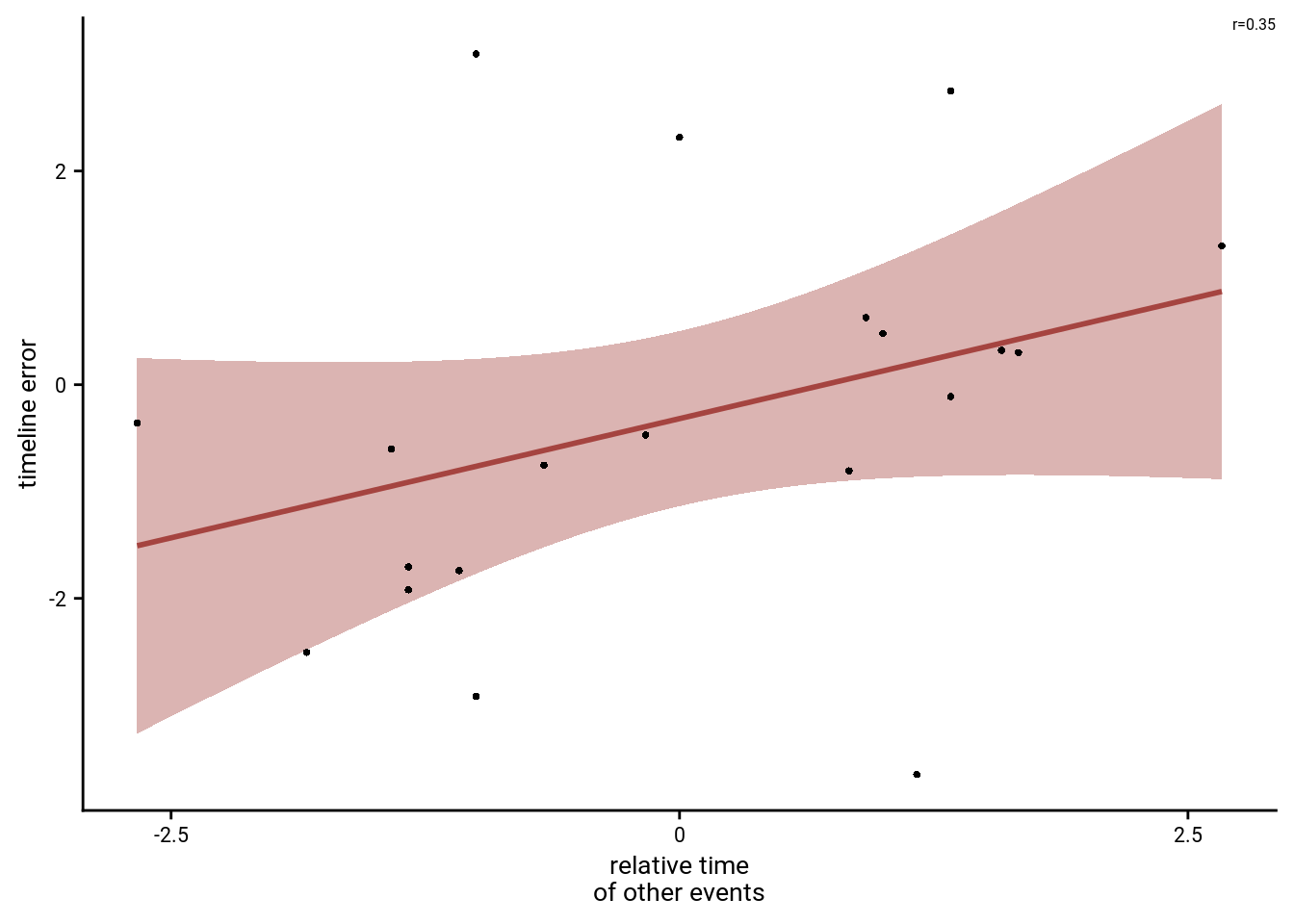Lastly, we show that the effect is quite visible from the single-subject plots: The slopes of the least-squares lines are, on average, positive.

# plot the relationship for each subject
sfig_bias_single_sub <- ggplot(beh_data, aes(x=rel_time_other_events, y=timeline_error)) +
geom_smooth(method='lm', formula= y~x,
color=aHPC_colors["across_main"],
fill=aHPC_colors["across_main"])+
geom_point(size = 1, shape = 16) +
facet_wrap(~sub_id, scales="free_y", nrow=4) +
scale_x_continuous(breaks = c(-2.5, 0, 2.5), labels= c("-2.5", "0", "2.5")) +
xlab("relative time of other events (virtual hours)") +
ylab("timeline error") +
theme_cowplot() +
theme(strip.background = element_blank(),
strip.text = element_blank(),
text = element_text(size=10, family=font2use),
axis.text = element_text(size=8))
sfig_bias_single_sub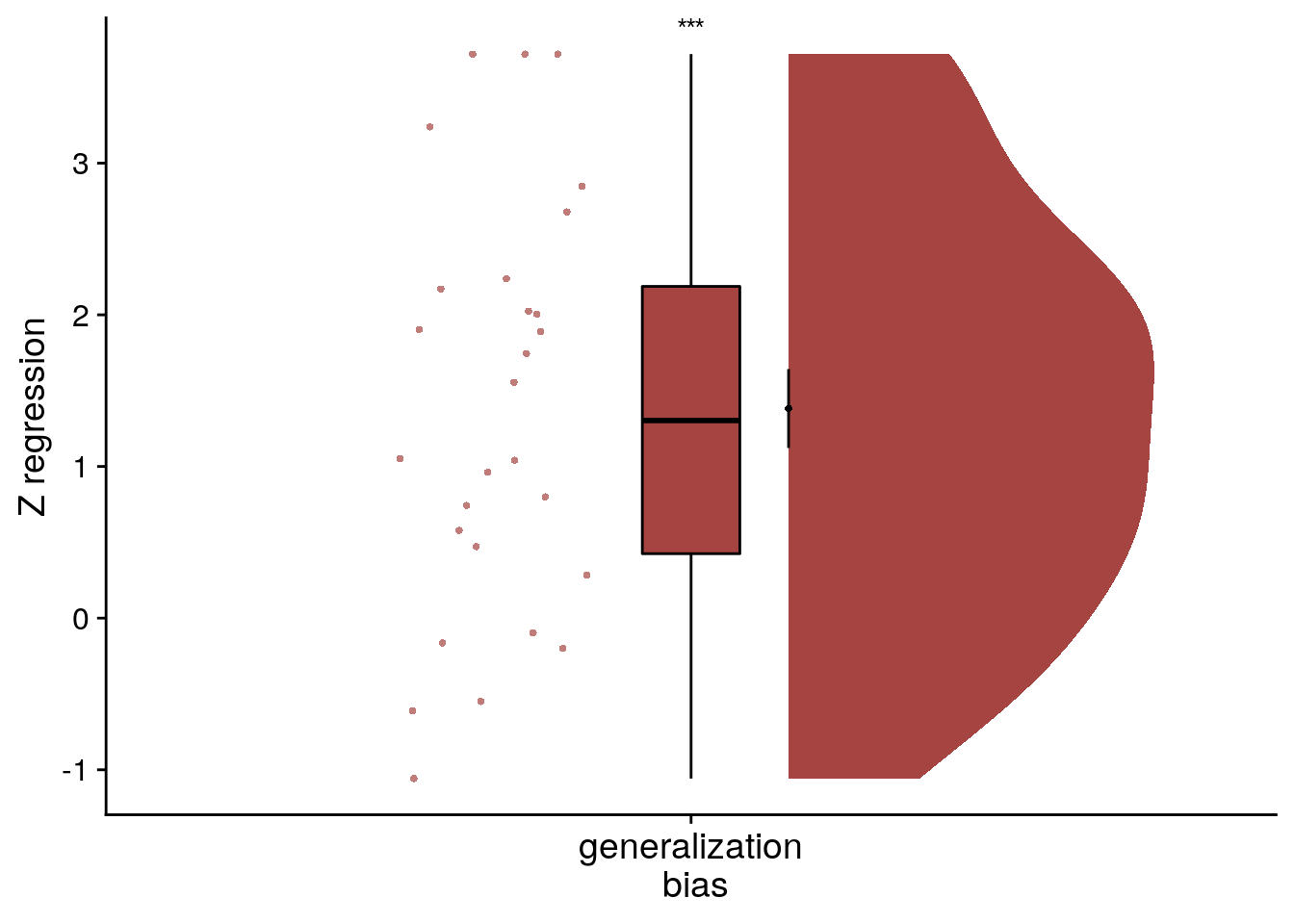## 5.4 Replication of Generalization Bias

To replicate the results from this exploratory analysis, we conducted the same analysis in an independent group of participants. These participants (n=46) constituted the control groups of a behavioral experiment testing the effect of stress induction on temporal memory (Montijn et al., 2021). They underwent the same learning task as described above with the only difference being the duration of this learning phase (4 rather than 7 mini-blocks of training). The timeline task was administered on the day after learning. The procedures are described in detail in Montijn et al. (2021). The data from this independent sample are shown in Figure 8F-H and Supplemental Figure 8B.

# load data from CSV
fname <- file.path(dirs$data4analysis, "beh_dataNDM.txt") col_types_list <- cols_only( sub_id = col_factor(), day = col_factor(), event = col_factor(), virtual_time = col_double(), memory_time = col_double() ) beh_data_replication <- as_tibble(read_csv(fname, col_types = col_types_list)) head(beh_data_replication) Table 5.7: sub_iddayeventvirtual_timememory_time P001119.259.21 P0011211.8 11.1 P0011314.8 16.1 P0011416.2 15 P0011518.2 18.4 P001219.5 8.5 ### Replication data: Quantify relative time of other events To test for such a bias, we quantify, for each event, the relative time of the other events at that sequence position. We then calculate by how much virtual time each individual event time deviates from the average virtual time of the other events at that sequence position (e.g. the difference in virtual time for event 1 from sequence 1 compared to the average virtual time of events 1 from sequences 2-4). We do this so that positive values of this relative time measure indicate that the other events happened later than the event of interest. # quantify the deviation in virtual time for each event relative to other events # at the same sequence position beh_data_replication <- beh_data_replication %>% add_column(rel_time_other_events=NA) for (i_day in 1:n_days){ for (i_event in 1:n_events_day){ # find the events at this sequence position from all four sequences curr_events <- beh_data_replication[beh_data_replication$event == i_event,]

# find the average virtual time of the other events at this sequence position
avg_vir_time_other_events <- curr_events %>%
filter(day != i_day) %>%
summarise(avg_vir_time = mean(virtual_time)) %>%
select(avg_vir_time) %>%
as.numeric(.)

# for the given event, store the relative time of the other events,
# as the difference in virtual time (positive values mean other events happen later)
event_idx <- beh_data_replication$day==i_day & beh_data_replication$event==i_event
beh_data_replication$rel_time_other_events[event_idx] <- avg_vir_time_other_events - beh_data_replication$virtual_time[event_idx]
}
}

### Replication data: Test Generalization Bias

The crucial test is then whether over- and underestimates of remembered time can be explained by this deviation measure.

# calculate timeline error so that positive numbers mean overestimates (later than true virtual time)
beh_data_replication <- beh_data_replication %>%
mutate(timeline_error=memory_time-virtual_time)

#### Replication data: Summary statistics

In the summary statistics approach, we run a linear regression model for each participant and test the resulting coefficients against a permutation-based null distribution. The resulting z-scores are then tested against 0 on the group level. Both of the tests rely on the custom stats function defined above.

# SUMMARY STATISTICS
set.seed(120) # set seed for reproducibility

# do RSA using linear model and calculate z-score for model fit from permutations
fit_beh_bias_replication <- beh_data_replication %>% group_by(sub_id) %>%
# run the linear model (also without permutation tests to get betas)
do(model = lm(timeline_error ~ rel_time_other_events, data=.),
z = lm_perm_jb(in_dat = .,
lm_formula = "timeline_error ~ rel_time_other_events",
nsim = n_perm)) %>%
# store beta estimates and their z-values
mutate(beta_rel_time_other_events = coef(summary(model))[2,"Estimate"],
t_rel_time_other_events = coef(summary(model))[2,"t value"],
z = list(setNames(z, c("z_intercept",  "rel_time_other_events")))) %>%
# get rid of intercept z-values and model column
unnest_longer(z) %>%
filter(z_id != "z_intercept") %>%
select (.,-c(model))

# run group-level t-tests on the RSA fits from the first level in aHPC for within-day
stats <- fit_beh_bias_replication %>%
filter(z_id =="rel_time_other_events") %>%
select(z) %>%
paired_t_perm_jb (., n_perm = n_perm)

# Cohen's d with Hedges' correction for one sample using non-central t-distribution for CI
d<-cohen.d(d=fit_beh_bias_replication$z, f=NA, paired=TRUE, hedges.correction=TRUE, noncentral=TRUE) ## Warning in pt(q = t, df = df, ncp = x): full precision may not have been achieved in 'pnt{final}' ## Warning in pt(q = t, df = df, ncp = x): full precision may not have been achieved in 'pnt{final}' stats$d <- d$estimate stats$dCI_low <- d$conf.int[] stats$dCI_high <- d\$conf.int[]

# print results
huxtable(stats) %>% theme_article()

Table 5.8:
estimatestatisticp.valuep_permparameterconf.lowconf.highmethodalternativeddCI_lowdCI_high
1.6611.39.76e-150.0001451.371.96One Sample t-testtwo.sided1.641.232.13
Summary Statistics: t-test against 0 for generalization bias in replication sample t45=11.3, p=0.000, d=1.64, 95% CI [1.23, 2.13]

We observe a significant positive effect of the virtual time of other events at the same sequence position on remembered virtual time. That means that when other events at the same sequence position are later than a given event, participants are likely to overestimate the event time. Conversely, when the other events at this sequence position relatively early, participants underestimate. This demonstrates an across-sequence effect of virtual time. Virtual time is generalized across sequences to bias remembered times at similar sequence positions.

To visualize this effect from the summary statistics approach, we create a raincloud plot of the z-values from the linear model permutations for each subject.

# plot regression z-values
f8f<-ggplot(fit_beh_bias_replication, aes(x=as.factor(1),y=z), colour= aHPC_colors["across_main"], fill=aHPC_colors["across_main"]) +
# plot the violin
gghalves::geom_half_violin(position=position_nudge(0.1),
side = "r", fill=aHPC_colors["across_main"], color = NA) +
geom_point(aes(x = 1-.2), alpha = 0.7,
position = position_jitter(width = .1, height = 0),
shape=16, colour=aHPC_colors["across_main"], size = 1)+
geom_boxplot(width = .1, fill=aHPC_colors["across_main"], colour = "black", outlier.shape = NA) +
stat_summary(fun = mean, geom = "point", size = 1, shape = 16,
position = position_nudge(.1), colour = "black") +
stat_summary(fun.data = mean_se, geom = "errorbar",
position = position_nudge(.1), colour = "black", width = 0, size = 0.5)+
ylab('Z regression') + xlab('generalization\nbias') +
annotate(geom = "text", x = 1, y = 3.7, label = "***", hjust = 0.5, size = 8/.pt, family=font2use) +
guides(color=FALSE, fill=FALSE)+
theme_cowplot() +
theme(axis.text.x = element_blank())
f8f#### Replication data: Mixed Model

We also want to this effect using a linear mixed model. We use the maximal random effects structure with random intercepts and random slopes for participants.

Let’s fit the model and get tidy summaries.

# z-score the relative time of other events for each participant
beh_data_replication <- beh_data_replication %>%
group_by(sub_id) %>%
mutate(rel_time_other_events_z = scale(rel_time_other_events)) %>%
ungroup()

# Fit the model with full random effect structure -> singular
lmm_full <- lme4::lmer("timeline_error ~ rel_time_other_events_z + (1+rel_time_other_events_z|sub_id)",
data=beh_data_replication, REML=FALSE)
## boundary (singular) fit: see ?isSingular
# fit again after removing correlation of slope and intercept
lmm_full <- lme4::lmer("timeline_error ~ rel_time_other_events_z + (1+rel_time_other_events_z||sub_id)",
data=beh_data_replication, REML=FALSE)
## boundary (singular) fit: see ?isSingular
# fit again after removing correlation of also random intercept (only random slopes left)
set.seed(278) # set seed for reproducibility
lmm_full <- lme4::lmer("timeline_error ~ rel_time_other_events_z + (0+rel_time_other_events_z|sub_id)",
data=beh_data_replication, REML=FALSE)
## boundary (singular) fit: see ?isSingular
# tidy summary of the fixed effects that calculates 95% CIs
lmm_full_bm <- broom.mixed::tidy(lmm_full, effects = "fixed", conf.int=TRUE, conf.method="profile")
## Computing profile confidence intervals ...
# tidy summary of the random effects
lmm_full_bm_re <- broom.mixed::tidy(lmm_full, effects = "ran_pars")

Compare against a reduced model without the fixed effect of interest.

# fit reduced model
set.seed(221) # set seed for reproducibility
lmm_reduced <- lme4::lmer("timeline_error ~ 1 + (0+rel_time_other_events_z|sub_id)",
data=beh_data_replication, REML=FALSE)
lmm_aov<-anova(lmm_full, lmm_reduced)
lmm_aov
Table 5.9:
nparAICBIClogLikdevianceChisqDfPr(>Chisq)
34.5e+03 4.52e+03-2.25e+034.5e+03
44.45e+034.47e+03-2.22e+034.44e+0353.712.29e-13

Mixed Model: Fixed effect of relative time of other events on timeline errors
$$\chi^2$$(1)=53.74, p=0.000

Make summary table.

fe_names <- c("intercept", "relative time other events")
re_groups <- c(rep("participant",1), "residual")
re_names <- c("relative time other events (SD)", "SD")

lmm_hux <- make_lme_huxtable(fix_df=lmm_full_bm,
ran_df = lmm_full_bm_re,
aov_mdl = lmm_aov,
fe_terms =fe_names,
re_terms = re_names,
re_groups = re_groups,
lme_form = gsub(" ", "", paste0(deparse(formula(lmm_full)),
collapse = "", sep="")),
caption = "Mixed Model: Behavioral generalization bias (replication)")

# convert the huxtable to a flextable for word export
stable_lme_beh_gen_bias_replication <- convert_huxtable_to_flextable(ht = lmm_hux)

# print to screen
theme_article(lmm_hux)
fixed effects term estimate SE t-value -0.320564 0.089155 -3.60 -0.495488 -0.145640 0.863631 0.091472 9.44 0.684152 1.043110 0.000000 2.704218 4501.04 -2247.52 4449.30 -2220.65 53.74 1 2.29e-13

To visualize the mixed model we create a dot plot of the fixed effect coefficient and the estimated marginal means.

# dot plot of Fixed Effect Coefficients with CIs
f8g <- ggplot(data = lmm_full_bm[2,], aes(x = term, color = term)) +
geom_hline(yintercept = 0, colour="black", linetype="dotted") +
geom_errorbar(aes(ymin = conf.low, ymax = conf.high, width = NA), size = 0.5) +
geom_point(aes(y = estimate), size = 1, shape = 16) +
scale_fill_manual(values = unname(aHPC_colors["across_main"])) +
scale_color_manual(values = unname(aHPC_colors["across_main"]), labels = c("across sequence bias")) +
labs(x = element_blank(), y="fixed\neffect estimate") +
theme_cowplot() +
theme(plot.title = element_text(hjust = 0.5), axis.text.x=element_blank()) +
guides(color=FALSE, fill=FALSE)+
annotate(geom = "text", x = 1, y = 1.1, label = "***", hjust = 0.5, size = 8/.pt, family=font2use)

# estimate marginal means for each model term by omitting the terms argument
lmm_full_emm <- ggeffects::ggpredict(lmm_full, ci.lvl = 0.95) %>% get_complete_df

# plot marginal means
f8h <- ggplot(data = lmm_full_emm, aes(color = group)) +
geom_line(aes(x, predicted)) +
geom_ribbon(aes(x, ymin = conf.low, ymax = conf.high, fill = group), alpha = .3, linetype=0) +
scale_color_manual(values = unname(aHPC_colors["across_main"]), name=element_blank()) +
scale_fill_manual(values = unname(aHPC_colors["across_main"])) +
scale_x_continuous(breaks = c(-2.5, 0, 2.5), labels= c("-2.5", "", "2.5")) +
ylab('estimated\nmarginal means') +
xlab('relative time\nof other events') +
guides(fill = FALSE, color=FALSE) +
theme_cowplot()
f8g+f8hLastly, we show that the effect is quite visible from the single-subject plots: The slopes of the least-squares lines are, on average, positive.

# plot the relationship for each subject
sfig_bias_replication_single_sub <- ggplot(beh_data_replication, aes(x=rel_time_other_events, y=timeline_error)) +
geom_smooth(method='lm', formula= y~x,
color=aHPC_colors["across_main"],
fill=aHPC_colors["across_main"])+
geom_point(size = 1, shape = 16) +
facet_wrap(~sub_id, scales="free_y", ncol=7) +
scale_x_continuous(breaks = c(-2.5, 0, 2.5), labels= c("-2.5", "0", "2.5")) +
xlab("relative time of other events (virtual hours)") +
ylab("timeline error") +
theme_cowplot() +
theme(strip.background = element_blank(),
strip.text = element_blank(),
text = element_text(size=10, family=font2use),
axis.text = element_text(size=8))

layout = "
A
A
A
A
B
B
B
B
B
B"

sfig_bias_single_sub_both_samples <- sfig_bias_single_sub + sfig_bias_replication_single_sub +
plot_layout(design = layout, guides = "keep") &
theme(text = element_text(size=10, family=font2use),
axis.text = element_text(size=8),
legend.text=element_text(size=8),
legend.title=element_text(size=8),
legend.position = "none"
) &
plot_annotation(tag_levels="A",
theme = theme(plot.margin = margin(t=0, r=0, b=0, l=-5, unit="pt")))

# save as png and pdf and print to screen
fn <- here("figures", "sf08")
ggsave(paste0(fn, ".pdf"), plot=sfig_bias_single_sub_both_samples, units = "cm",
width = 17.4, height = 22.5, dpi = "retina", device = cairo_pdf)
ggsave(paste0(fn, ".png"), plot=sfig_bias_single_sub_both_samples, units = "cm",
width = 17.4, height = 22.5, dpi = "retina", device = "png")
sfig_bias_single_sub_both_samples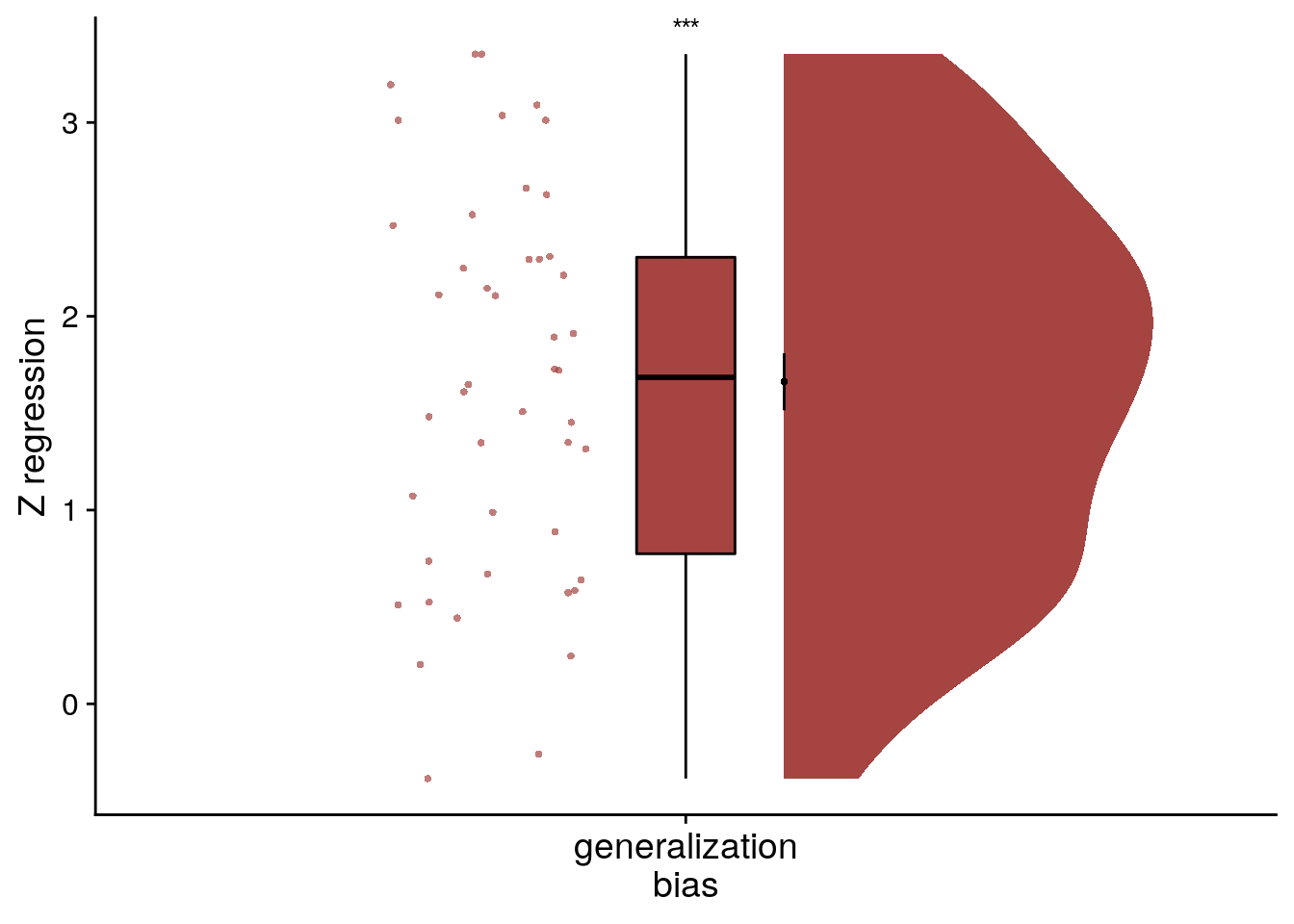Supplemental Figure 8. Generalization bias in individual participants. A, B. Each panel shows the data from one participant. Each circle corresponds to one event. The x-axis indicates the average relative time of the events occupying the same sequence position in other sequences. The y-axis shows the signed error of constructed event times as measured in the timeline task. The regression line and its confidence interval are overlaid in red. Positive slopes of the regression line indicate that constructed event times are biased by the average time of events in the other sequences. A shows data from the main sample; B from the replication sample.